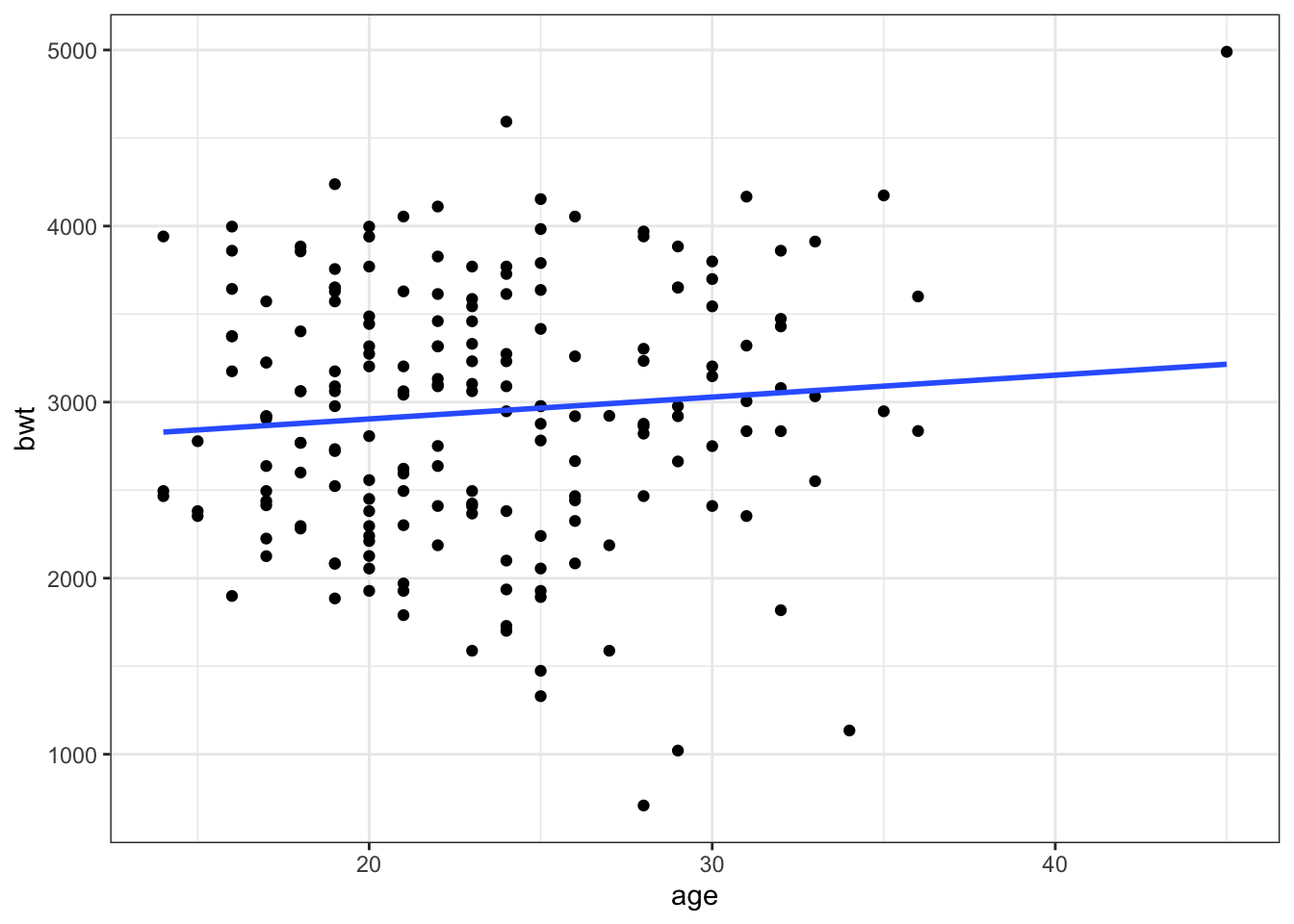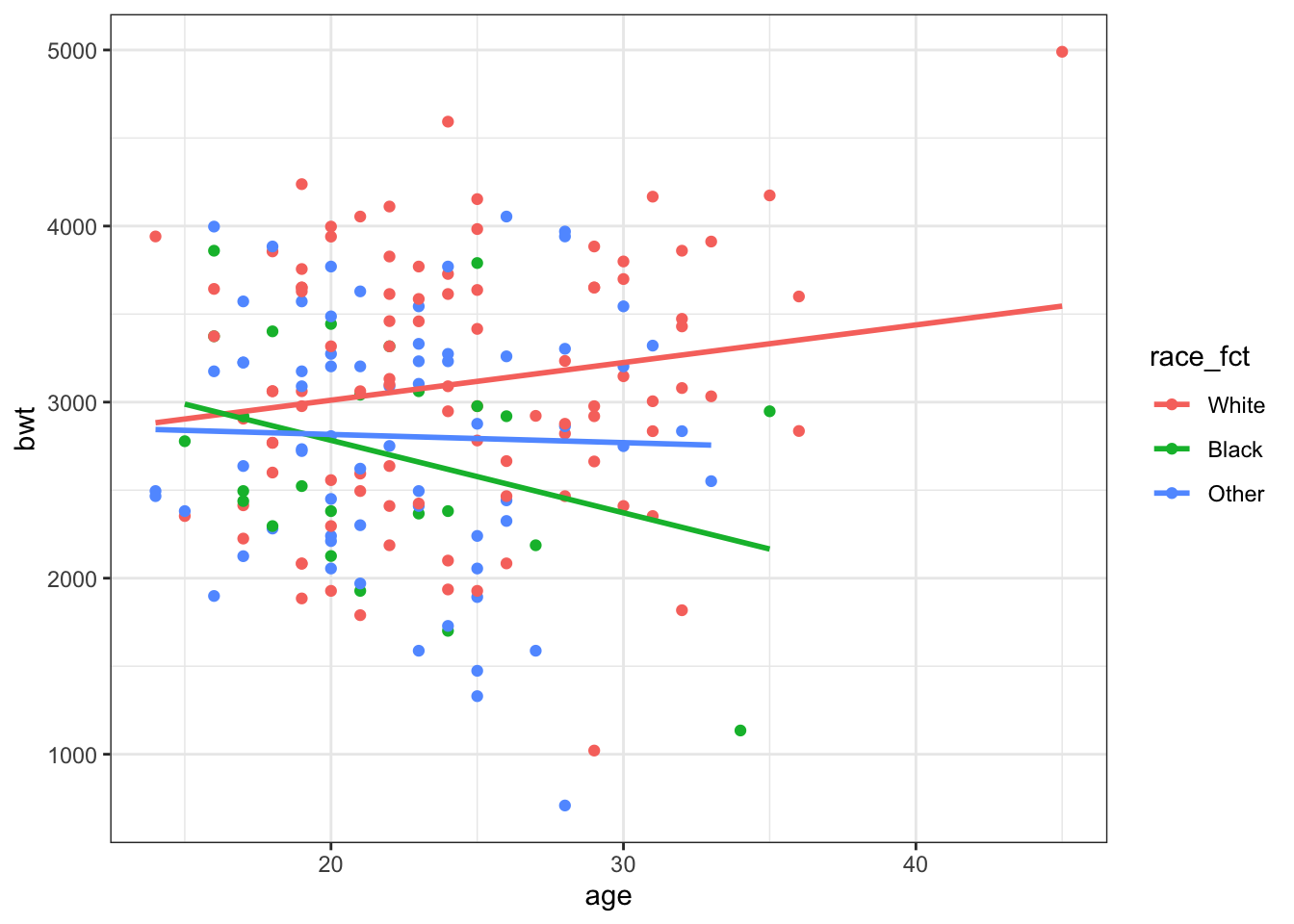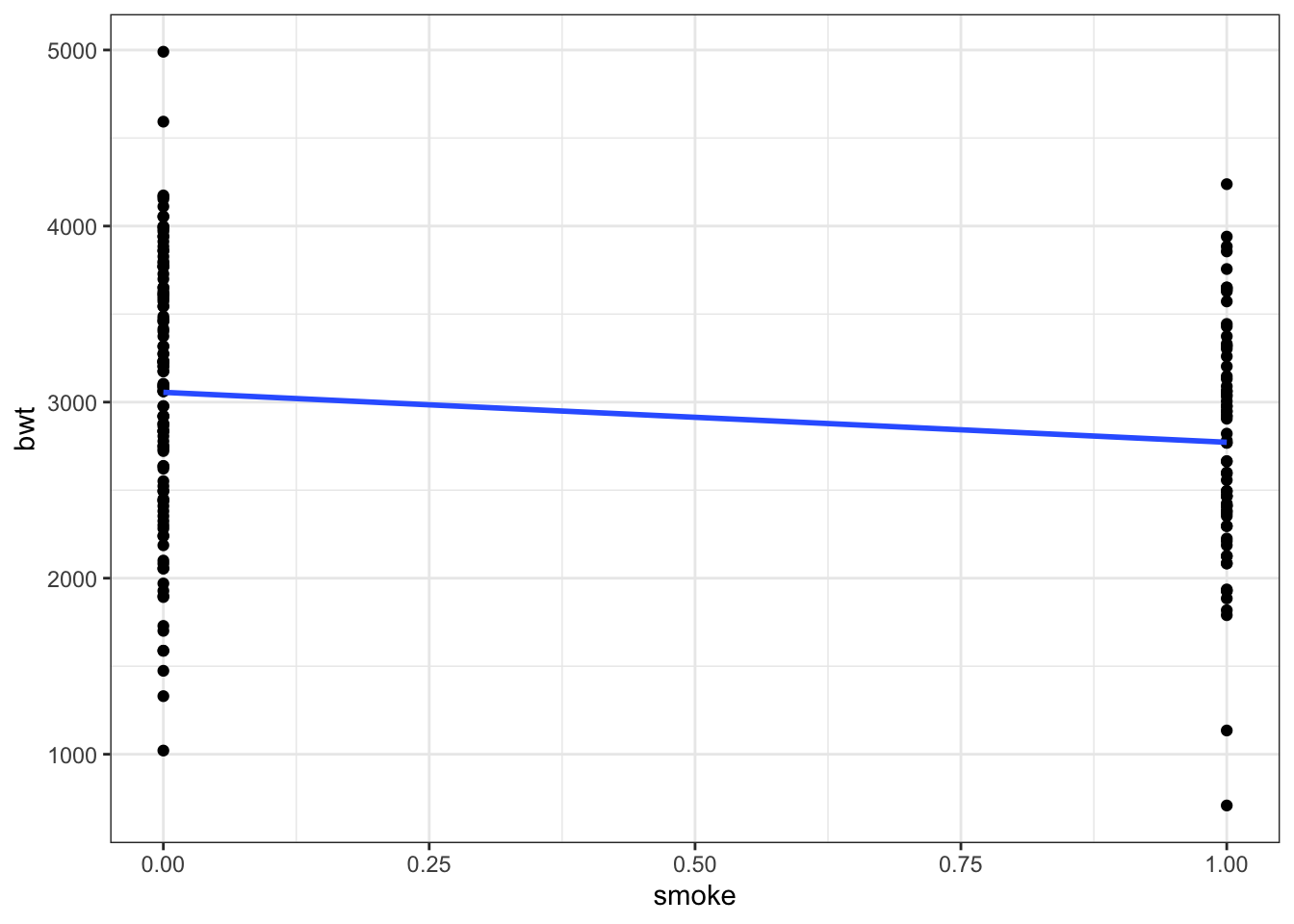# Model fit and out of sample prediction

Using birthweight data from the MASS library we will work through evaluating different modeling specifications:

library(MASS) # birthwt dataset
library(texreg) # for nice looking tables
library(tidyverse)
## Warning: package 'tibble' was built under R version 3.6.2
## Warning: package 'dplyr' was built under R version 3.6.2

We will use the birthweight data to explore different model specifications. Here, bwt is the outcome variable of interest, birthweight.

head(birthwt)
##    low age lwt race smoke ptl ht ui ftv  bwt
## 85   0  19 182    2     0   0  0  1   0 2523
## 86   0  33 155    3     0   0  0  0   3 2551
## 87   0  20 105    1     1   0  0  0   1 2557
## 88   0  21 108    1     1   0  0  1   2 2594
## 89   0  18 107    1     1   0  0  1   0 2600
## 91   0  21 124    3     0   0  0  0   0 2622

The race variable is mother’s race and is coded:

• 1: White
• 2: Black
• 3: Other

birthwt <- birthwt %>%
mutate(race_fct = recode(race, 1 = 'White', 2 = 'Black', 3 = 'Other'))
# specify factor variable with White as reference category
birthwt$race_fct <- factor(birthwt$race_fct, levels = c('White', 'Black', 'Other'))
#check variable
table(birthwt$race, birthwt$race_fct)
##
##     White Black Other
##   1    96     0     0
##   2     0    26     0
##   3     0     0    67

Let’s explore what some of the predictor variables look like.

ggplot(data = birthwt, aes(x = age, y = bwt)) +
geom_point() +
geom_smooth(method=lm,se=F) +
theme_bw()
## geom_smooth() using formula 'y ~ x'Figure 1: Age Plot

ggplot(data = birthwt, aes(x = age, y = bwt, color = race_fct)) +
geom_point() +
geom_smooth(method=lm,se=F) +
theme_bw()
## geom_smooth() using formula 'y ~ x'Figure 2: Age and Race Plot

The initial Figure 1 seems to suggest there is not much relationship (or maybe slightly positive) between age and birthweight. However, when we look at Figure 2, age by race, there seems to be different relationships across groups suggesting an interation between these predictors.

ggplot(data = birthwt, aes(x = smoke, y = bwt)) +
geom_point() +
geom_smooth(method=lm,se=F) +
theme_bw()
## geom_smooth() using formula 'y ~ x'ggplot(data = birthwt, aes(x = smoke, y = bwt, color= race_fct)) +
geom_point() +
geom_smooth(method=lm,se=F) +
theme_bw()
## geom_smooth() using formula 'y ~ x'Looking at the relationship between smoking and birthweight in general there seems to be a negative relationship. But again, there might be an interactive effect with race.

Let’s compare different linear model specifications:

m1 <- lm(bwt ~ age + smoke, data = birthwt)
m2 <- lm(bwt ~ age + smoke + race_fct, data = birthwt)
htmlreg(list(m1,m2),doctype = F)
Statistical models
Model 1 Model 2
(Intercept) 2791.22*** 3281.67***
(240.95) (260.66)
age 11.29 2.13
(9.88) (9.77)
smoke -278.36* -426.09***
(106.99) (109.99)
race_fctBlack -444.07**
(156.19)
race_fctOther -447.86***
(119.02)
R2 0.04 0.12
Num. obs. 189 189
RMSE 717.19 690.02
p < 0.001, p < 0.01, p < 0.05

Including race of the mother, which we know from the health disparities literature in the United States is substantively important, is also statistically significant. In addition, we see that that the adjusted R squared has increased and the root mean squared error (RMSE) has decreased.

m3 <- lm(bwt ~ age*race_fct + smoke, data = birthwt)
m4 <- lm(bwt ~ age*race_fct + smoke*race_fct, data = birthwt)
htmlreg(list(m1,m2,m3,m4),doctype = F)
Statistical models
Model 1 Model 2 Model 3 Model 4
(Intercept) 2791.22*** 3281.67*** 3032.07*** 3251.05***
(240.95) (260.66) (338.74) (353.34)
age 11.29 2.13 11.60 6.83
(9.88) (9.77) (12.85) (12.98)
smoke -278.36* -426.09*** -389.78*** -580.08***
(106.99) (109.99) (113.67) (146.58)
race_fctBlack -444.07** 394.58 256.56
(156.19) (699.09) (705.20)
race_fctOther -447.86*** -61.30 -332.36
(119.02) (541.85) (554.01)
age:race_fctBlack -37.46 -39.59
(30.69) (32.12)
age:race_fctOther -15.87 -11.43
(22.74) (22.70)
race_fctBlack:smoke 365.94
(336.12)
race_fctOther:smoke 522.09*
(263.26)
R2 0.04 0.12 0.13 0.15
Adj. R2 0.03 0.10 0.10 0.11
Num. obs. 189 189 189 189
RMSE 717.19 690.02 690.68 686.31
p < 0.001, p < 0.01, p < 0.05

Based on the adjusted R squared and RMSE it looks like including age and smoke as interactions with race make for the best fitting model.

While, including additional predictors can improve model fit it can also lead to overfitting. One way to approach this is to separate your data into a training set and a test set. Use the training set to fit the model and then look at how well the model works on the out-of-sample (test) data. A model that is not overfit will continue to perform well.

Usually, we would want to randomly separate the data into a training and test set.

set.seed(12345)
# randomly draw row numbers 80% for training set, 20% for test set
train_obs <- sample(x=1:nrow(birthwt),size=.8*nrow(birthwt),replace = F)
train <- birthwt[train_obs,]
test <- birthwt[-train_obs,]

However, in order to demonstrate how overfitting can be a problem I’m going to separate into a very small training set.

train_obs <- c(1,2,3,29,42,57,140,152,138,165,167,184)
train <- birthwt[train_obs,]
test <- birthwt[-train_obs,]

Now, we fit our models on the training data and choose our preferred model.

m_train <- lm(bwt ~ age*race_fct + smoke*race_fct, data = train)
m_train1 <- lm(bwt ~ age + smoke, data = train)
m_train2 <- lm(bwt ~ age + smoke + race_fct, data = train)
htmlreg(list(m_train, m_train1, m_train2),doctype = F)
Statistical models
Model 1 Model 2 Model 3
(Intercept) 17145.00 2156.67* 2174.26*
(16560.35) (772.11) (842.86)
age -767.00 9.48 5.44
(871.30) (33.09) (37.22)
race_fctBlack -15246.07 -23.62
(16684.66) (393.45)
race_fctOther -15860.41 243.91
(16652.40) (397.39)
smoke 752.00 192.37 182.26
(1444.88) (301.00) (328.89)
age:race_fctBlack 776.91
(876.18)
age:race_fctOther 809.27
(873.55)
race_fctBlack:smoke -260.96
(1571.43)
race_fctOther:smoke 58.11
(1679.96)
R2 0.52 0.04 0.12
Num. obs. 12 12 12
RMSE 616.10 501.27 546.38
p < 0.001, p < 0.01, p < 0.05

We make a judgment using our normal diagnostic tools like adjusted R squared, RMSE, etc. This example is slightly unrealistic because of the very small sample size but let’s choose the interactive model. This model has an R squared of 0.52 but the adjusted R squared is negative because of the very small sample size. We should be wary that a model fit on only 12 observations is overfitting.

What is the mean of the squared residuals?

obs_y_train <- train$bwt pred_y_train <- m_train$fitted.values
resid_train <- obs_y_train - pred_y_train
mean(resid_train^2)
##  94894.57
# compare this to resid stored in summary
mean(m_train$residuals^2) ##  94894.57 RMSE_train <- sqrt(mean(m_train$residuals^2))
RMSE_train
##  308.0496

Now, we will use the estimated coefficients from this model to predict birthweight on the test data.

# predict by hand
betas_train <- as.matrix(summary(m_train)$coefficients[,1]) # We need the dimensions of the testing data to match our betas test <- test %>% mutate(race_fctBlack = ifelse(race_fct=='Black',1,0), race_fctOther = ifelse(race_fct=='Other',1,0), age:race_fctBlack = age*race_fctBlack, age:race_fctOther = age*race_fctOther, race_fctBlack:smoke = race_fctBlack*smoke, race_fctOther:smoke = race_fctOther*smoke) # remove outcome variable and needs to be a matrix X <- test %>% select(age,race_fctBlack, race_fctOther, smoke, age:race_fctBlack,age:race_fctOther, race_fctBlack:smoke,race_fctOther:smoke) %>% as.matrix() # add column of 1s for intercept X <- cbind(1, X) colnames(X) <- "(Intercept)" #add column name to intercept In order to use matrix multiplication the dimensions must be compatible. # check dimensions dim(X) ##  177 9 dim(betas_train) ##  9 1 Estimate the predicted birthweights using the coefficients from the training model but the data from the test dataset. pred_y_test <- X %*% betas_train resid_test <- test$bwt - pred_y_test
mean(resid_test^2)
##  21549599
RMSE_test <- sqrt(mean(resid_test^2))
RMSE_test
##  4642.155

The mean of the squared residuals and therefore RMSE have increased meaning the model does not fit as well on this data.

We can also use the built-in predict() function to do the same things:

newdata <- test %>% select(bwt, age, smoke, race_fct)
pred_y_test2 <- predict(m_train, newdata = newdata)
mean((test\$bwt - pred_y_test2)^2)
##  21549599

This gives us the same results and saves us from having to create a compatable dataset.

Let’s fit a new model on the test data and compare (normally we would not do this. The test data is held out and only used for validation of the model).

m_test <- lm(bwt ~ age*race_fct + smoke*race_fct, data = test)
m_all <- lm(bwt ~ age*race_fct + smoke*race_fct, data = birthwt)
htmlreg(list(m_train,m_test,m_all),doctype=F,custom.model.names = c("Train", "Test", "All"))
Statistical models
Train Test All
(Intercept) 17145.00 3433.18*** 3251.05***
(16560.35) (365.72) (353.34)
age -767.00 1.38 6.83
(871.30) (13.28) (12.98)
race_fctBlack -15246.07 142.48 256.56
(16684.66) (739.12) (705.20)
race_fctOther -15860.41 -494.33 -332.36
(16652.40) (586.32) (554.01)
smoke 752.00 -611.83*** -580.08***
(1444.88) (150.93) (146.58)
age:race_fctBlack 776.91 -32.58 -39.59
(876.18) (33.93) (32.12)
age:race_fctOther 809.27 -6.26 -11.43
(873.55) (24.18) (22.70)
race_fctBlack:smoke -260.96 282.47 365.94
(1571.43) (372.95) (336.12)
race_fctOther:smoke 58.11 529.44 522.09*
(1679.96) (282.21) (263.26)
R2 0.52 0.16 0.15